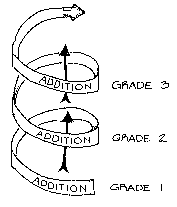### Activating Inappropriate Existing Knowledge

Nicole McNeil of the Department of Psychology at Notre Dame published a paper in Child Development about ten years ago entitled "Limitations to Teaching Children 2 + 2 = 4: Typical Arithmetic Problems". She offers a different title on a version of this article at Notre Dame: "Don't Teach Children 2 + 2". Of course, it is widely known that arithmetic fluency correlates with good performance in algebra. For instance, a study involving about 200 undergraduate students concludes "computational fluency had the strongest effect on algebra achievement". So, why should we not teach children arithmetic? This recommendation is actually more about how knowledge can sometimes hinder learning.

McNeil, in a much more recent work with other researchers, highlights the importance of understanding math equivalence, that is, appreciating what an equal sign really entails. To assess how well a student understand math equivalence, questions like the one shown below can be used:

67 + 84 = _?_ + 83

Finding the answer to the above does not require simply performing an arithmetic operation. It is not just adding 67 and 84 since the sum, 151, is not the correct answer. The correct answer is 68. Providing 151 as the answer by young children illustrates one of the challenges children face in studying math equivalence. In addition, they often miss where the equal sign is. For instance, in 3 + 9 + 5 = 6 + _, students sometimes give 23 (which is 3 + 9 + 5 + 6) as the answer. These mistakes suggest that children are unable to leave the simple problems they have encountered when learning arithmetic. It does seem that children are stuck on one specific type of problem: Doing one mathematical operation to get to the answer.

McNeil's older paper provides insights on why children may have this particular challenge. By performing two experiments, in which one group of students are taught math equivalence while another group is taught arithmetic as an additional lesson on top of math equivalence, McNeil demonstrates that tapping into existing knowledge that is quite narrow compared to what one is learning is detrimental. Children who are given arithmetic exercises in between lessons on math equivalence perform poorly in the math equivalence tests than those who are not given arithmetic problems to practice or review.

This reminds me of something I posted on this blog years ago:"If we do not think spirally, why do we have to learn or teach spirally? And this is what makes it so difficult. There are many right words in the quote and the right intentions. But, as long as the fundamentals are wrong, the pedagogy behind the spiral approach is not viable. Concepts - classes - are not organized in a single hierarchy. Rather there is a web of related classes in which a variety of hierarchies can be distinguished. Recognizing individual hierarchies is as important as discerning relationships between the classes. But spirals?" Figure and caption taken from http://www.cut-the-knot.org/Mset99/examples.shtml
"American schools follow a "spiral curriculum" in mathematics; that is, they spend such a substantial proportion of time on review each year that only limited progress can be made with new material…. American students who perform poorly in arithmetic are subject to a special form of the spiral curriculum, which might be termed the circular curriculum": they repeat arithmetic over and over until they stop studying math" (Gamoran, 2001, p. 138)"

Gamoran, A. (2001). Beyond curriculum wars: Content and understanding in mathematics. In T. Loveless, Ed., The Great Curriculum Debate, pp. 134-162. Washington, D.C.: Brookings Institution Press.